Oxidation Reduction Reactions

·       Oxidation is loss of electrons

·       Reduction is gain of electrons

Activity series of metals

·       Metal ions will replace ions of a less easily oxidized metal from solution
Cd+2  +  Fe  ®  Fe +2  +   Cd

Redox reactions can be expressed as the sum of two  half reactions

·       Cd +2   + 2  e - ®   Cd   and

·       Fe      ®   Fe +2  +  2 e -                        Note electrons must cancel

Suppose we do  Cd +2   +  Fe  where the product is Fe +3

Write the half reactions, balance the number of electrons, and add:

Organic compounds also undergo redox reactions:

·       { C H2O }  + O2   ®   CO2   +  H2O

How do we know that the oxidation states have changed here?

Rules for oxidation numbers:

·       uncombined elements have an oxidation state of 0

·       H in compounds is usually +1except in hydrides of Group 1 and 2 metals where it is -1 (CaH2, for example)

·       O in compounds is usually -2 (except in peroxides)

·       Group 1 metals are +1 in compounds, and Group 2 metals are +2

·       F is always -1 in compounds

·       The sum of all the oxidation states of the component atoms must equal the total charge on the molecule or ion.

So for our reaction

What are the oxidation state changes?

MnO4-  +   Fe+2   +  H+   ®   Mn+2   +   Fe+3   +  H2O

Oxidation states?

Half reactions are:

NH4+  +  2 O2  ®  NO3-   +  2 H+  +  H2O

An important oxidation of ammonia from breakdown of organic waste to nitrate which is useful for plant growth

Oxidation numbers?

What is oxidized?

What is reduced?

What is the oxidizing agent?

The reducing agent?

Important redox reactions are catalyzed by microorganisms

Also, different reactions may occur depending on whether the reaction is taking place in acid or basic solution.

Oxidation reaction can be separated in space from the reduction reaction, if there is a way for the electrons and ions to be exchanged:

Fe +3    +      H2    ®      Fe +2    +       H+

Can be carried out in a cell ( 2 half cells  )

H2   Û   2 H+  + 2 e-                                                E0  =  0 V

and

Fe +3    +   e -     Û     Fe +2                                   E0  = +0.77 V

Standard Hydrogen Electrode:  H+ at 1 M,  H2 at 1 atm is arbitrarily assigned a potential of 0.00 V.

At standard concentrations, in this system hydrogen is oxidized and Fe +3 is reduced. Electrons flow from the Fe half cell to the H half cell.

Potential measured in the wire is the difference between the potentials of the two half reactions. In this case, 0.77 V is measured when all concentrations are standard.
The cell voltage can be expressed as volts or as the electron activity, analogous to pH. Use pE or pE0 instead of E, or E0.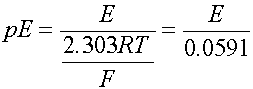when T is 298 oK, or 25 oC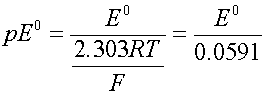where R is the gas constant (8.314 J/mol deg) and F is the Faraday constant (96500 coul/mol).

It is also defined as pE = - log (ae-)

but the electron activity can only be measured as a voltage difference between two half cells.

But voltage does change with concentration

The Nernst Equation is used to calculate this change: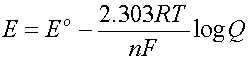where n is the number of electrons involved in the reaction

and Q is the reaction quotient (same form as the K)

i.e: for the reaction    Fe +3  +  e-   Û  Fe +2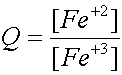and        n = 1

As defined, when all concentrations are 1,

then Q = 1/1 =1 and log Q = 0 and E = E0

Can divide each term by 2.303RT/F to get the Nernst equation in terms of pE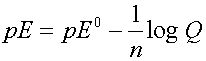Half reactions can be added to give complete reactions and pE0 values are used to determine the direction of reaction.

2 H+  +  2 e-  ®  H2     pE0 = 0.0

Pb +2  +  2 e-  ®  Pb     pE0 = -2.13

If we connect two half cells, one with a standard H2 electrode, and one with a lead electrode in a 1 M Pb +2 solution, a potential will be generated. Lead will dissolve from its electrode. The potential will start out as the difference between the two half cell potentials: 0.0 V - (-2.13)  = 2.13 V

Corresponding to the two half reactions:

2 H+ + 2 e- ® H2     pE0 = 0.0

Pb  ®  Pb +2 + 2 e-  pE0 = - (-2.13 )

2 H+  +  Pb  ®   H2  + Pb +2

Writing the Nernst eqn for this reaction gives: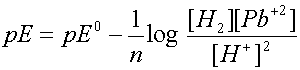What happens if we connect the two cells and let the reaction go??
As reaction proceeds Q ® Keq, and pE ® 0

When the reactions reach equilibrium Q = Keq  and  pE =  0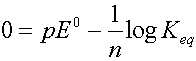So:          log Keq  =  n (pE0)

D G, the Gibbs Free Energy Change

D G is the free energy of a reaction- the decrease in G in a reaction is the maximum amount of work which can be done by the reaction. If DG is negative, the reaction is spontaneous.

Also can be calculated from the cell voltage:

DG = -2.303nRT(pE)

if everything is in the standard state then

DGo = -2.303nRT(pEo)

DGo can be calculated from tabulated thermodynamic data, so actual cell measurements are not needed to find Eo values.

What can we find from DG?

Keq, Eo, spontaneity of a reaction,

Can we put an electrode and a std H2 reference electrode in water and measure pE, as we do for pH? Actually, very difficult to get accurate results. (LJP chief source of problems, esp. because E’s in water are pretty small, as strong oxidizing or reducing situations don’t usually happen )

Limits of pE in Water

·             pE indicates the activity (concentration) of  electrons in the water

·             A high pE means ???

·             A low pE means???

When the activity of e- is very low, species which can be oxidized will tend to lose electrons

When the activity of e- is very high, species which can be reduced will tend gain electrons

·             The pE is limited in water by the reactions:

2 H2O  Û O2  +  4H+ +  4 e-         and

2 H2O  +  2 e-   Û  H2   +   2OH-   or      H+  +  e-   Û  1/2 H2

for the first reaction --from the table pE0  = 20.75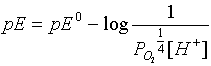and if pO2 is 1 atm, then

pE = 20.75  - pH

So the oxidizing limit of water depends on pH  (as we suspect from the H+ in tne reaction!)

For the reducing limit

H+  +  e-   Û  1/2 H2                   pE  =  0.0

pE  =  0.0  -  log 1/[H+]     or     pE  =  -  pH

These give the theoretical boundaries of the pE in water, but because of slow kinetics it can sometines exist outside these limits

What are the pE limits in pH 7 water?
Can also calculate the pE in water under various conditions:

When saturated with air at neutral pH:

(PO2)  =  .21 atm  and  pH = 7

When water is saturated with methane and CO2 as it would be in an anerobic condition:

P CO2  =  P CH4  and pH = 7

·                   E0  or pE0  is an intensive property--does NOT depend on how much material is present or the size of the cell or if we write it for one electron or many!

·                   K does depend on how we write the equation:
eg:

·                   H2  +  1/2 O2  ®   H2O

or

·                   2 H2  +  O2  ®  2 H2O

but in the Nernst equation, the change in n makes the E stay the same.